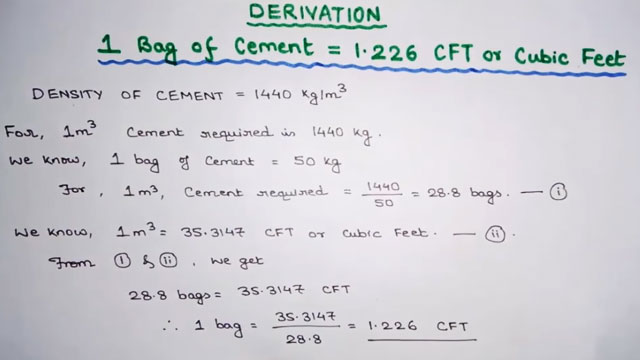## Density Of Cement Formula | Volume Of Cement Bag In Cubic Meters

This construction video is recorded on the detailed process for obtaining the quantity of 1.226 cft cement in 1 bag.

Here density of cement is taken as 1400 kg per m3 (m = millimeter)

Therefore, the quantity of cement for 1m3 = 1440 Kg

It is assumed 1 bag cement = 50 kg
Therefore, cement required for 1m3 = 1440/50 = 28.8 bags (This is taken as equation 1)
It is assumed 1m3 = 35.3147 CFT (Cubic Feet) (It is taken as equation 2)

By combining both equation, the following result is obtained :-

28.8 bags similar to 35.3147 CFT

Therefore, 1 bag is similar to 35.3147/28.8 = 1.226 CFTsource